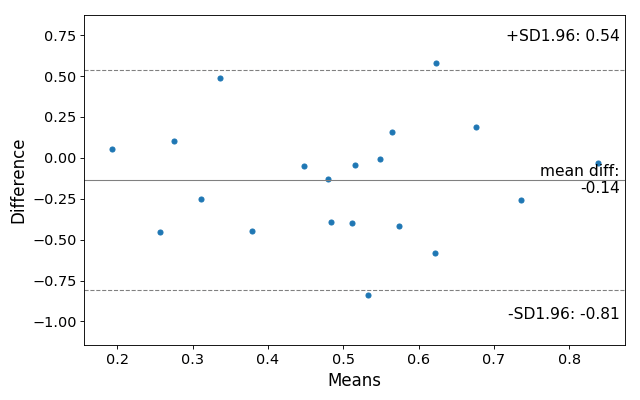/Statsmodels

# statsmodels.graphics.agreement.mean_diff_plot

`statsmodels.graphics.agreement.mean_diff_plot(m1, m2, sd_limit=1.96, ax=None, scatter_kwds=None, mean_line_kwds=None, limit_lines_kwds=None)` [source]

Tukey’s Mean Difference Plot.

Tukey’s Mean Difference Plot (also known as a Bland-Altman plot) is a graphical method to analyze the differences between two methods of measurement. The mean of the measures is plotted against their difference.

For more information see https://en.wikipedia.org/wiki/Bland-Altman_plot

Parameters: m2 (m1,) – sd_limit (float, default 1.96) – The limit of agreements expressed in terms of the standard deviation of the differences. If `md` is the mean of the differences, and `sd` is the standard deviation of those differences, then the limits of agreement that will be plotted will be md - sd_limit * sd, md + sd_limit * sd The default of 1.96 will produce 95% confidence intervals for the means of the differences. If sd_limit = 0, no limits will be plotted, and the ylimit of the plot defaults to 3 standard deviatons on either side of the mean. ax (matplotlib AxesSubplot instance, optional) – If `ax` is None, then a figure is created. If an axis instance is given, the mean difference plot is drawn on the axis. scatter_kwargs (keywords) – Options to to style the scatter plot. Accepts any keywords for the matplotlib Axes.scatter plotting method mean_line_kwds (keywords) – Options to to style the scatter plot. Accepts any keywords for the matplotlib Axes.axhline plotting method limit_lines_kwds (keywords) – Options to to style the scatter plot. Accepts any keywords for the matplotlib Axes.axhline plotting method fig – If `ax` is None, the created figure. Otherwise the figure to which `ax` is connected. matplotlib Figure

#### References

Bland JM, Altman DG (1986). “Statistical methods for assessing agreement between two methods of clinical measurement”

#### Example

Load relevant libraries.

```>>> import statsmodels.api as sm
>>> import numpy as np
>>> import matplotlib.pyplot as plt
```

Making a mean difference plot.

```>>> # Seed the random number generator.
>>> # This ensures that the results below are reproducible.
>>> np.random.seed(9999)
>>> m1 = np.random.random(20)
>>> m2 = np.random.random(20)
>>> f, ax = plt.subplots(1, figsize = (8,5))
>>> sm.graphics.mean_diff_plot(m1, m2, ax = ax)
>>> plt.show()
```© 2009–2012 Statsmodels Developers
© 2006–2008 Scipy Developers
© 2006 Jonathan E. Taylor
Licensed under the 3-clause BSD License.
http://www.statsmodels.org/stable/generated/statsmodels.graphics.agreement.mean_diff_plot.html